Technical Information Site of Power Supply Design

2016.11.24 Transfer Function

# Amplifier Virtual Short

DC/DC Converters: Sharing of Transfer Functions among Control Systems

In the previous section, transfer functions for an error amplifier and for a voltage amplifier and a current amplifier were derived. In this section, we consider the virtual short method, which is often used as a means of determining amplifier transfer functions.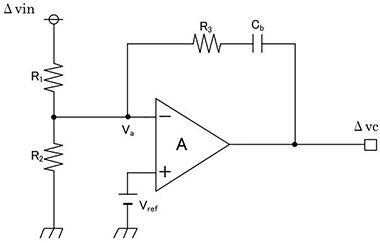Fig.4

Amplifier virtual short

When studying operational amplifiers and examining their various characteristics, an ideal op-amp and a virtual short (also called an imaginary short) are important. Similarly, the method of a virtual short is widely used when determining the transfer functions of amplifiers.

In deriving a transfer function, by regarding Va in Fig. 4 as Vref and a virtual short, grounding is assumed for small signals, and the equation Δa = 0 is used.

The transfer function derived using this method for Fig. 4 is indicated in eq. 2-9.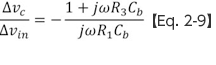Fig. 5 shows the gain and phase in the Bode diagram obtained using this equation (solid lines) and in the Bode diagram obtained by actual measurements (dashed lines).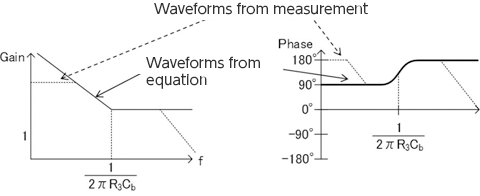Fig. 5

From Fig. 5, the difference between the characteristics represented by the equation and measurements can be discerned.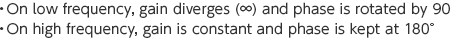These results mean that "there are conditions for a region in which a virtual short can be applied".
In order for an ideal virtual short to be valid, the following conditions must be met.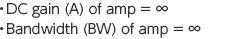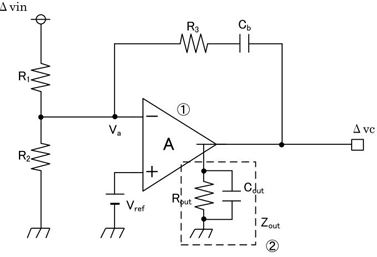Fig. 6

However, these conditions are not actually satisfied. In actuality, the DC gain and bandwidth are both limited by circuit characteristics such as transistor gm values and the output impedance, as indicated in Fig. 6.

As a result, the following are obtained.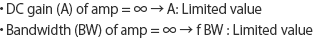This means that on the low-frequency side, the DC gain is limited, and on the high-frequency side the band is limited and therefore the gain is decreased. Let's examine how this phenomenon affects the transfer function.

If C1=0, then the previous eq. 2-9, derived assuming a virtual short, and eq. 2-6 derived in the previous section using Kirchhoff's laws, become similar equations. However, eq. 2-6 was derived assuming eq. 2-5 as a precondition. This is the condition for a virtual short.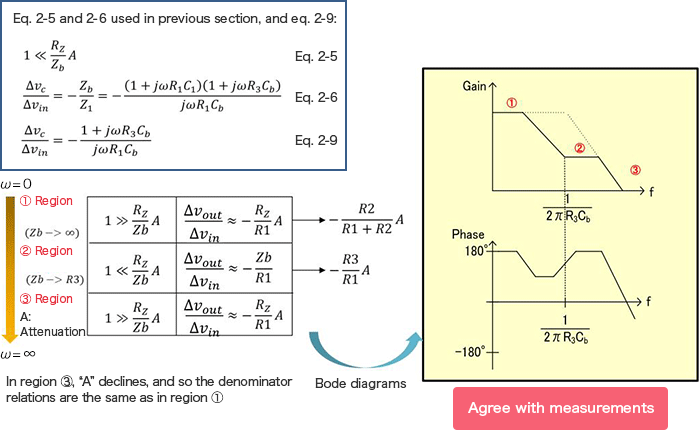Fig. 7

Now let's consider the effect of deviations from ideal conditions due to the characteristics described above in relation to eq. 2-5.

On the low-frequency side (ω = 0), with A limited, eq. 2-5 becomes

Zb → ∞, RsA → limited value

and the condition does not obtain.

On the high-frequency side (ω = ∞), when A≒0,

Zb → R3、RsA → 0

and again the condition does not obtain.
Hence for an actual circuit, the transfer function changes according to the frequency region as in Fig. 7.

Finally, application of the virtual short method is summarized in Fig. 8. When deriving an amplifier transfer function, it should be understood that there are regions in which the a virtual short method can and cannot be applied, and an appropriate method of derivation must be used for each region.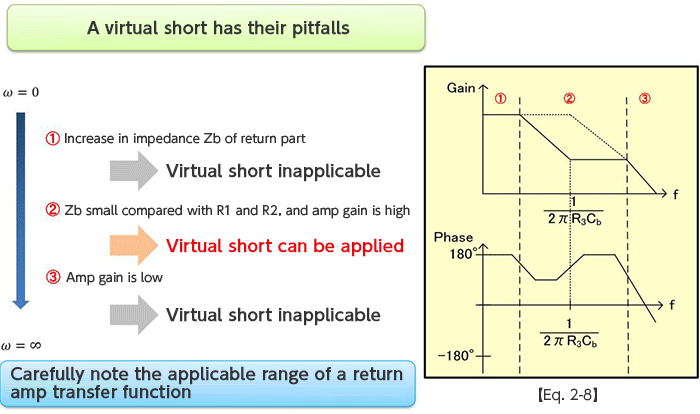Fig. 8